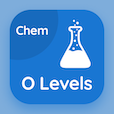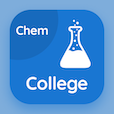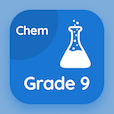Cambridge Online Courses (GCSE)

O Level Chemistry Quizzes

O Level Chemistry Quiz - Complete

Learn Chemical formulas quiz questions, chemical formulas MCQ with answers PDF, test 40 to learn O Level Chemistry online course. Chemical Formulae and Equations trivia questions, chemical formulas Multiple Choice Questions (MCQ Quiz) for online college degrees. Chemical formulas Book PDF: valency and chemical formula, molecules and macromolecules, chemical reaction: factor affecting, strong and weak acids, chemical formulas test prep for GRE test.

"The chemical formula of monohydrate monosodium phosphate is" Quiz PDF: chemical formulas App APK with po2nah2o, h2onapo2, nah2po4.h2o, and nah3po2.h2o choices for SAT prep classes. Study chemical formulae and equations questions and answers to improve problem solving skills for online degree programs.

## Quiz on Chemical formulas MCQs

MCQ: The chemical formula of monohydrate monosodium phosphate is

H2ONaPO2
PO2NaH2O
NaH2PO4.H2O
NaH3PO2.H2O

MCQ: Weak acids include

H2CO3 only
H2SO3 only
H2SO4
H2CO3 and H2SO3

MCQ: Suitable apparatus to measure the volume of gas given off is a

tap funnel
conical beefle
gas syringe

MCQ: A shared pair of electrons like Cl2 forms

ionic bonds
single covalent bond
double covalent bond
dative bond

MCQ: The chemical formula of aluminum oxide is

Al2O3
AlO4
Al2O5.H2O
Al2O5

### More Quizzes from O Level Chemistry Book